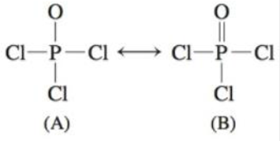# Complete the following resonance structures for POCl 3 . a. Would you predict the same molecular structure from each resonance structure? b. What is the hybridization of P in each structure? c. What orbitals can the P atom use to form the π bond in structure B? d. Which resonance structure would be favored on the basis of formal charges?### Chemistry: An Atoms First Approach

2nd Edition
Steven S. Zumdahl + 1 other
Publisher: Cengage Learning
ISBN: 9781305079243

#### Solutions

Chapter
Section### Chemistry: An Atoms First Approach

2nd Edition
Steven S. Zumdahl + 1 other
Publisher: Cengage Learning
ISBN: 9781305079243
Chapter 4, Problem 94AE
Textbook Problem
187 views

## Complete the following resonance structures for POCl3.a. Would you predict the same molecular structure from each resonance structure?b. What is the hybridization of P in each structure?c. What orbitals can the P atom use to form the π bond in structure B?d. Which resonance structure would be favored on the basis of formal charges?

(a)

Interpretation Introduction

Interpretation: The various questions based upon the resonance structure of POCl3 are to be answered.

Concept introduction: When the atomic orbitals overlap with each other in the region where density of electrons is high, then molecular orbitals are formed. Overlap of the atomic orbitals determines the efficiency of the interaction between the atomic orbitals.

Energy of bonding molecular orbitals is less than the nonbonding molecular orbitals.

To determine: If the molecular structure of each of the resonance structures is same or not.

### Explanation of Solution

Explanation

The molecular structure of each of the resonance structures is same.

The molecular structure of each of the resonance structures is tetrahedral...

(b)

Interpretation Introduction

Interpretation: The various questions based upon the resonance structure of POCl3 are to be answered.

Concept introduction: When the atomic orbitals overlap with each other in the region where density of electrons is high, then molecular orbitals are formed. Overlap of the atomic orbitals determines the efficiency of the interaction between the atomic orbitals.

Energy of bonding molecular orbitals is less than the nonbonding molecular orbitals.

To determine: The hybridization of phosphorus in both the structure.

(c)

Interpretation Introduction

Interpretation: The various questions based upon the resonance structure of POCl3 are to be answered.

Concept introduction: When the atomic orbitals overlap with each other in the region where density of electrons is high, then molecular orbitals are formed. Overlap of the atomic orbitals determines the efficiency of the interaction between the atomic orbitals.

Energy of bonding molecular orbitals is less than the nonbonding molecular orbitals.

To determine: The orbitals used by phosphorus to form pi bond in structure B.

(d)

Interpretation Introduction

Interpretation: The various questions based upon the resonance structure of POCl3 are to be answered.

Concept introduction: When the atomic orbitals overlap with each other in the region where density of electrons is high, then molecular orbitals are formed. Overlap of the atomic orbitals determines the efficiency of the interaction between the atomic orbitals.

Energy of bonding molecular orbitals is less than the nonbonding molecular orbitals.

To determine: The resonance structure favored that is favorable on the basis of formal charges.

### Still sussing out bartleby?

Check out a sample textbook solution.

See a sample solution

#### The Solution to Your Study Problems

Bartleby provides explanations to thousands of textbook problems written by our experts, many with advanced degrees!

Get Started

Find more solutions based on key concepts
Most of the time, the buyer is wasting his or her money when buying an ergogenic aid to improve performance. St...

Nutrition: Concepts and Controversies - Standalone book (MindTap Course List)

What causes an aging giant star to produce a planetary nebula?

Horizons: Exploring the Universe (MindTap Course List)

What is an immunoglobulin?

Organic And Biological Chemistry

Review. As shown in Figure P10.77, two blocks are connected by a string of negligible mass passing over a pulle...

Physics for Scientists and Engineers, Technology Update (No access codes included)

What is lithification? How is sedimentary rock formed?

Oceanography: An Invitation To Marine Science, Loose-leaf Versin